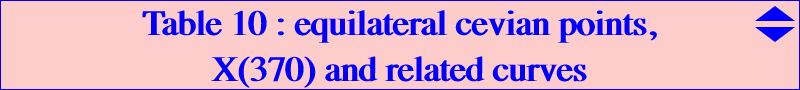This page has been written using several insightful ideas by Jean-Pierre Ehrmann A point P is an equilateral cevian point if the cevian triangle of P is equilateral (TCCT, p.267). The corresponding center of the triangle is denoted by Q. There are 6 such points P which lie on the Neuberg cubic: 4 are always real, one of them being X(370), the only one inside ABC. Naturally, there are also 6 corresponding points Q which lie on the Napoleon cubic and on the perpendicular bisectors L1 and L2 of X(13)X(15) and X(14)X(16) respectively. Those on L2 (blue corresponding points P ) are always real but those on L1 (red corresponding points P) are not necessarily : the always real point on L1 is associated to X(370). Each line through P and the corresponding Q passes through O. See also Q033. These 6 points P (and their isogonal conjugates P*) lie on a large number of curves which are shown below. See also table 14 and table 40 when "cevian" is replaced with "anticevian" and "circumanticevian".Conics and circles passing through the equilateral cevian pointsTwo groups of three conics through the points P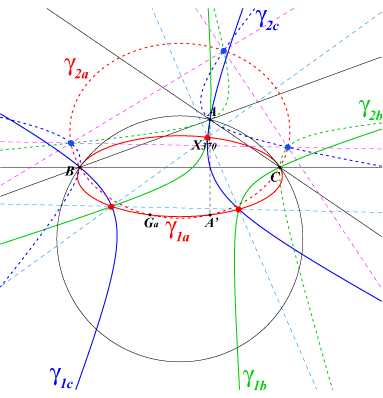gamma_1a and gamma_2a are the conics with equations : Sqrt3[S_B y(x+z)+S_C z(x+y)] - 2S x(y+z+2x) = 0 Sqrt3[S_B y(x+z)+S_C z(x+y)] + 2S x(y+z+2x) = 0 which pass through B, C, A' (reflection of A in BC), Ga (S is the area of ABC). The lines passing through A' and the third vertices of the two equilateral triangles erected externally on AB, AC meet the lines AB, AC at two points on gamma_1a. For gamma_2a, replace externally by internally. The remaining four conics are constructed similarly. The conics gamma_1x contain the red points, and the conics gamma_2x the blue points.A group of two conics through the points P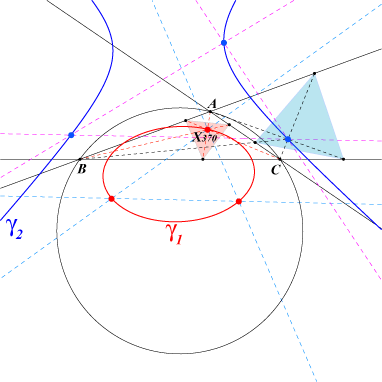gamma_1 is the "sum" of the three conics gamma_1x seen above. Its equation is : Sqrt3 (C) - 2S (G) = 0 where : (C) = a^2yz + cyclic (G) = x^2 + yz + cyclic showing that gamma_1 belongs to the pencil of conics generated by the circumcircle and an imaginary ellipse with center G, homothetic of the Steiner ellipse. gamma_1 contains the red points and X616. Similarly, gamma_2 has equation : Sqrt3 (C) + 2S (G) = 0, and contains the blue points and X617. In other words, the conics gamma_1x generate a net N_1 of conics which contains gamma_1. The figure shows two of the six equilateral cevian triangles.A group of two circles through the points P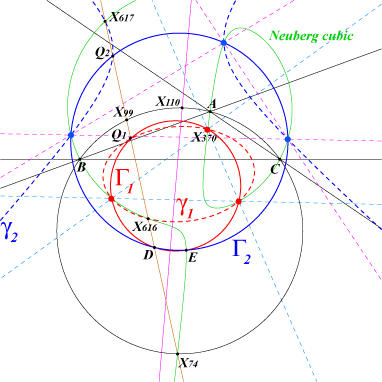The net N_1 seen above also contains a circle which is Gamma_1. Gamma_2 is defined similarly. These two circles pass through D (reflection of H in X110) and E (reflection of D in the line X20-X110). Their centers Ω1, Ω2 lie on this line X20-X110. D is X(12383) in ETC and E is X(5667) = X(30)-Ceva conjugate of X(4). Gamma_1 and gamma_1 meet at the red points and at Q1. Gamma_2 and gamma_2 meet at the blue points and at Q2. Q1 and Q2 lie on the line X74-X99. The equations are rather complicated. Click on the link to download a text file of ten conics mentioned above :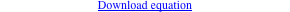A group of two rectangular hyperbolas through the points P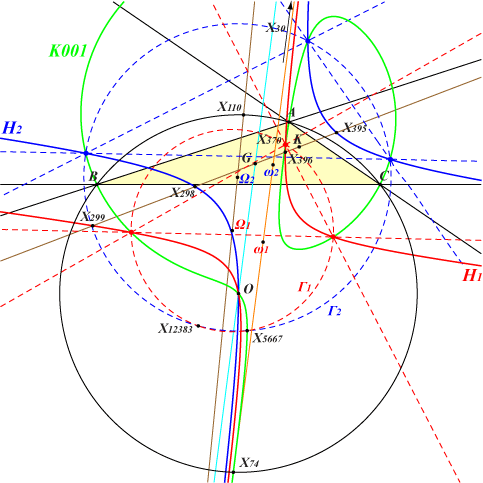The red points (including X370) lie on the rectangular hyperbola H1, with center ω1, passing through X(3), X(30), X(299), X(396), X(523). The blue points lie on the rectangular hyperbola H2, with center ω2, passing through X(3), X(30), X(298), X(395), X(523). These two centers lie on the line X30-X74 which is the real asymptote of the Neuberg cubic K001.A group of two circles and a group of two rectangular hyperbolas through the points P*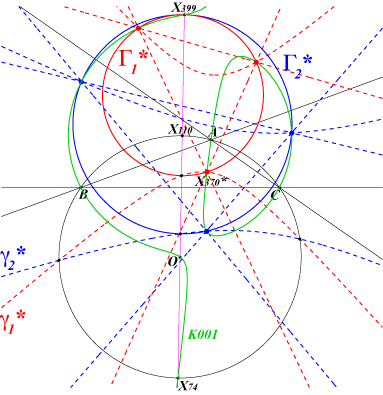Similarly, it is not difficult to find analogous groups of six conics, two conics and two circles passing through the isogonal conjugates P* of the 6 points P. These points P* obviously also lie on the Neuberg cubic. We only show the group of circles Gamma_1*, Gamma_2* and the group of conics gamma_1*, gamma_2* (beware these curves are NOT the isogonal conjugates of the ones met before). Gamma_1*, Gamma_2* are both centered on the line through O, X74, X110, X399 (this latter point lying on both circles). Gamma_1* and gamma_1* intersect at the isogonal conjugates of the red points and meet again at a point on the line O-X110. Similarly, Gamma_2* and gamma_2* intersect at the isogonal conjugates of the blue points and also meet again at a point on the line O-X110. Note that gamma_1* and gamma_2* meet on the circumcircle.Click on the link to download a text file of all ten conics here mentioned.The isogonal conjugates of the red points lie on the rectangular hyperbola passing through X(4), X30), X(396), X(523), X(3181). The isogonal conjugates of the blue points lie on the rectangular hyperbola passing through X(4), X30), X(395), X(523), X(3180). Both hyperbolas have their center on the line X30-X74.Cubics passing through the equilateral cevian pointsThe table below sums up different cubics through these red and blue points P :cubic name points other points K001 Neuberg cubic P, P, P*, P* see the Neuberg page K143-A-B-C X(370)-cubics P, P Ga, Gb, Gc K144 X(370)-antimedial cubic P, P X(2), X(3), X(30), X(5667), Ga, Gb, Gc K264a pK(X2, X298) P X(2), X(13), X(298), X(616) K264b pK(X2, X299) P X(2), X(14), X(299), X(617) K265 X(370)-medial cubic P, P midpoints K266 X(370)-equilateral cubic P, P K341a pK(X15, X2) P X(2), X(3), X(6), X(13), X(15), X(18), X(298), X(470), X(1082), X(2992), X(5240) K341b pK(X16, X2) P X(2), X(3), X(6), X(14), X(16), X(17), X(299), X(471), X(559), X(2993), X(5239) K419a pK(X13, X11078) P X(2), X(13), X(14), X(30), X(298), X(395), X(472), X(1081) K419b pK(X14, X11092) P X(2), X(13), X(14), X(30), X(299), X(396), X(473), X(554), X(7043)Remark 1 : there are two cubics which are both decomposed into three lines, each passing through either the red or blue points P. They are shown in the figures above in dashed lines. The equations are complicated. Remark 2 : K264a, K341a, K419a belong to a same pencil of circum-cubics through the blue points. This pencil contains no other pK and three very complicated and not always real nK0s. The same remark applies to K264b, K341b, K419b and the red points.Higher degree curves passing through the equilateral cevian pointsThe table below sums up different higher degree curves through these red and blue points P :curve name points other points Q033 X(370)-quartic P, P X(2), X(3), X(30) Q034 X(370)-Fermat quintic P, P X(2), X(3), X(13), X(14) Q035-A-B-C X(370)-central quartics P, P Ga, Gb, Gc Q036-A-B-C X(370)-central quintics P, P Ga, Gb, Gc Q074 X(370)-Soddy quintic P, P X(2), X(4), Soddy centers, X(616), X(617), etc Q099 X(370)-quartic P, P X(3), X(6), X(1249) Q100 Dergiades septic P, P X(2), X(7), X(369), etc Q105 Montesdeoca septic P, P X(1), X(2), X(3), X(1138), etc Q111 a quintic P, P X(3), X(4), X(1263), X(2992), X(2993)